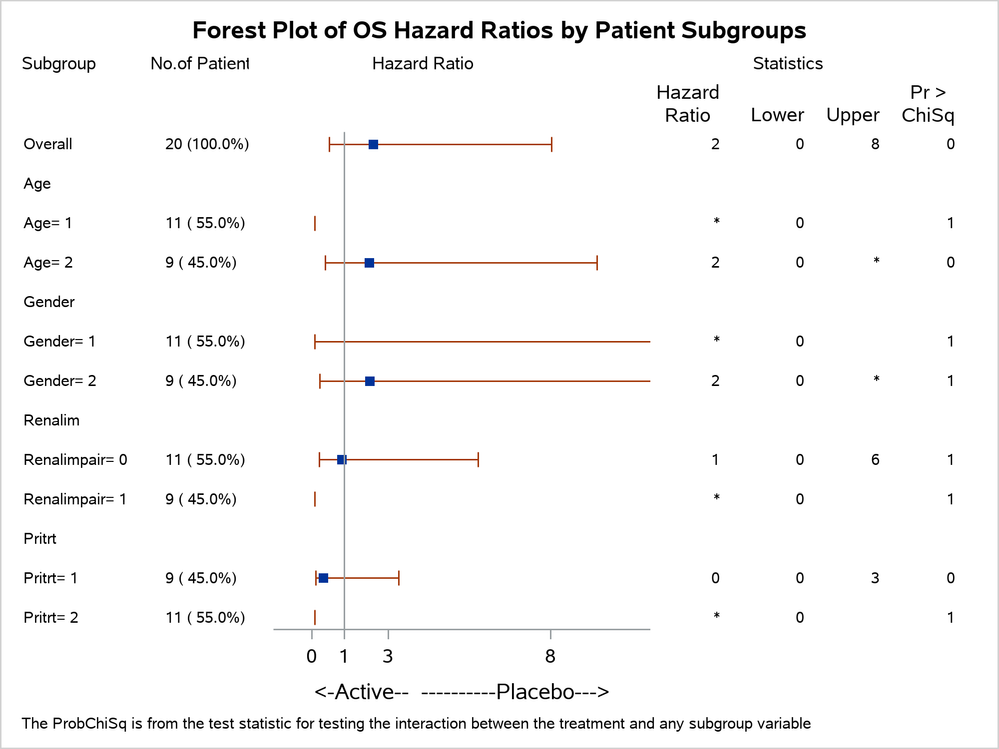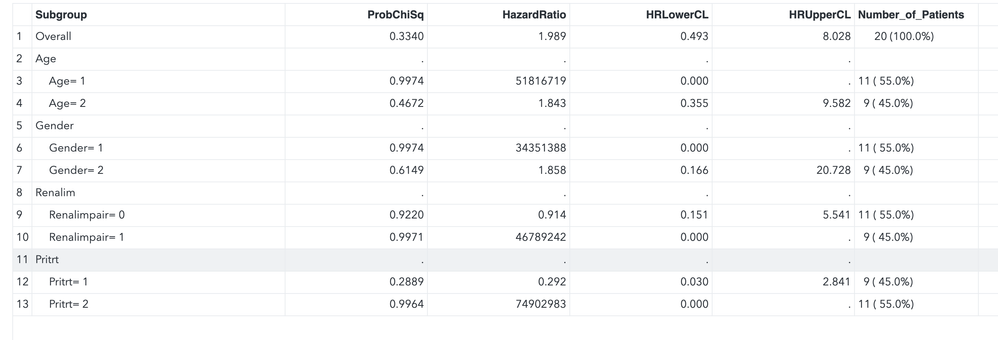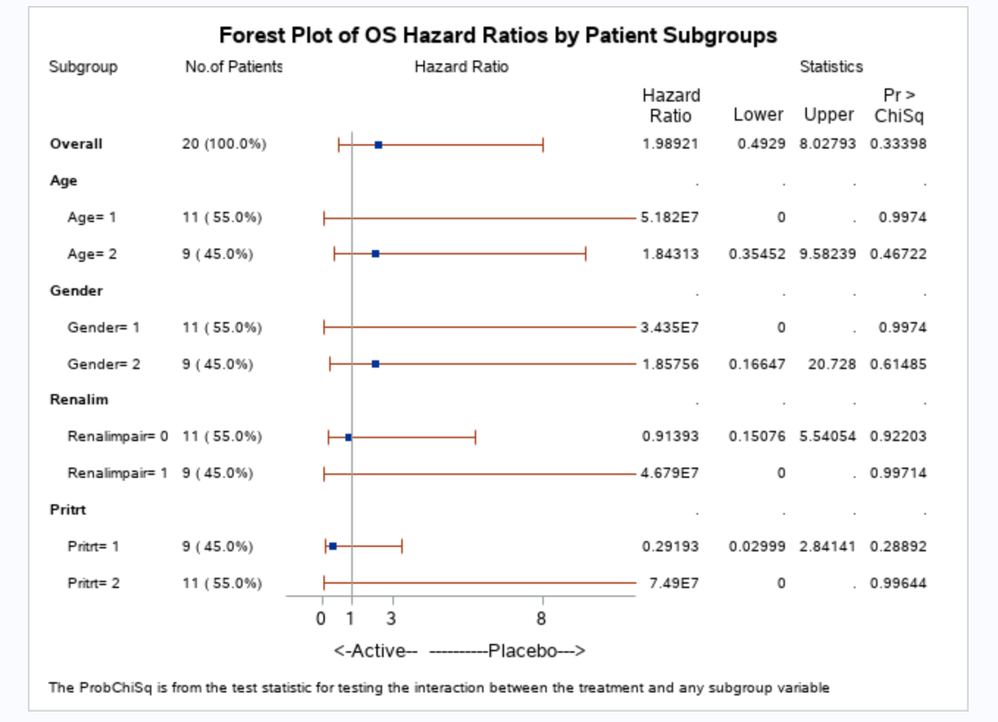## Numbers show in star (*) in proc sgrender

Hi guys,

When I was using proc template and sgrender to get a forest plot. The statistic numbers round themself or show as a star(*) if the number is very large.  As you can see in attached file.

Any thoughts?

Thanks,

Ray1 ACCEPTED SOLUTION

Accepted Solutions

## Re: Numbers show in star (*) in proc sgrender

Specify a VALUEFORMAT= options for variables to control appearance such as

```***********4th Column*****************;
layout overlay / x2axisopts=(display=none offsetmin=0.25 offsetmax=0.25 )
yaxisopts=(reverse=true display=none) walldisplay=none;

axistable y=subgroup value=HazardRatio valueformat=6.4 ;
endlayout;```

to display the Hazard ration with 6 print positions and 4 decimals.

If you aren't sure what format you want you might try BEST6. for all the problem columns to see what gets displayed and adjust as desired.

6 REPLIES 6

## Re: Numbers show in star (*) in proc sgrender

The single most common cause of such a display I have found is specifying a format that displays too few characters for the value.

If you run this code the log will display an * as you mention:

```data example;
x=10;
put x= f1.;
run;```

The reason is there is no way for a value such as 10 or greater to be displayed with a single character (unless you go to HEX and then you have a limit of 16 base 10). Values less than 10 can be rounded to a single digit in some fashion but no way for larger.

So you likely need to change the format you are using but I can't suggest one without knowing the ranges of values and what you would like to display.

If you share your template or sgrender code we might suggest where to make a format change.

## Re: Numbers show in star (*) in proc sgrender

Thank you for your help. I post my dataset and code below. I used 8.3 format for my dataset(all those numeric variables).Here is my code. Problem column is the 4,5,6 and 7th column as I comment below.

roc template;
define statgraph Ray;
dynamic _show_bands _color _thk inserttitle;
begingraph;
entrytitle 'Forest Plot of' inserttitle 'Hazard Ratios by Patient Subgroups';

discreteattrmap name='text';
value '0' / textattrs=(weight=bold);
value other;
enddiscreteattrmap;
discreteattrvar attrvar=type var=indent attrmap='text';

layout lattice / columns=7 columnweights=(0.15 0.12 0.4 0.1 0.08 0.08 0.09);

sidebar / align=top;
layout lattice / rows=1 columns=4 columnweights=(0.15 0.12 0.4 0.35);
entry textattrs=(size=8) halign=left "Subgroup";
entry textattrs=(size=8) halign=left " No.of Patients";
entry textattrs=(size=8) halign=center "Hazard Ratio";
entry halign=center textattrs=(size=8) "Statistics" ;
endlayout;
endsidebar;

********First group**********;
layout overlay / walldisplay=none xaxisopts = (display=none)
yaxisopts = (reverse=true display=none tickvalueattrs=(weight=bold));
/* referenceline y = subgroup / lineattrs=(thickness=_thk color=_color); */
axistable y=subgroup value=subgroup /indentweight=indent textgroup=type
display=(values);
endlayout;

**********Second column***********;
layout overlay / walldisplay=none xaxisopts = (display=none)
yaxisopts = (reverse=true display=none tickvalueattrs=(weight=bold));
/* referenceline y = subgroup / lineattrs=(thickness=_thk color=_color); */
axistable y=subgroup value=Number_of_Patients /display=(values);
endlayout;
***********Third column**************;
layout overlay / xaxisopts=(label='<-Active-- ----------Placebo--->'
linearopts=(viewmin=-1 viewmax=11
tickvaluelist=(-0.1 0.0 0.5 1.0 1.5 2.0 2.5 3.5 5.0 8.0)))
yaxisopts=(reverse=true display=none) walldisplay=none;
*referenceline y=user_label / lineattrs=(thickness=_thk color=_color);
scatterplot y=subgroup x=hazardratio / xerrorlower=hrlowercl xerrorupper=hruppercl
markerattrs=(symbol=squarefilled);
referenceline x=1;
endlayout;

***********4th Column*****************;
layout overlay / x2axisopts=(display=none offsetmin=0.25 offsetmax=0.25 )
yaxisopts=(reverse=true display=none) walldisplay=none;

axistable y=subgroup value=HazardRatio ;
endlayout;
******5th Column********;
layout overlay / x2axisopts=(display=(tickvalues) offsetmin=0.25 offsetmax=0.25)
yaxisopts=(reverse=true display=none) walldisplay=none;
axistable y =subgroup value = HRLowerCL ;
endlayout;

******6th Column********;
layout overlay / x2axisopts=(display=(tickvalues) offsetmin=0.25 offsetmax=0.25)
yaxisopts=(reverse=true display=none) walldisplay=none;
axistable y =subgroup value = HRUpperCL ;
endlayout;

******7th Column********;
layout overlay / x2axisopts=(display=(tickvalues) offsetmin=0.25 offsetmax=0.25)
yaxisopts=(reverse=true display=none) walldisplay=none;
axistable y =subgroup value = ProbChiSq;
endlayout;

endlayout;

entryfootnote halign=left textattrs=(size=7)
'The ProbChiSq is from the test statistic for testing the interaction between the treatment and any subgroup variable';
endgraph;
end;
run;

proc format;
value misblank
.='';
run;

ods html file = 'figure_Forest.html' image_dpi = 300 style = htmlblue;
ods graphics on / reset imagename = 'figure_Forest' outputfmt = png;
proc sgrender data=forest template=ray;
format probchisq hazardratio hrlowercl hruppercl misblank.;
dynamic _color='cxf0f0f' _thk=12 inserttitle = ' OS ';
run;

## Re: Numbers show in star (*) in proc sgrender

Specify a VALUEFORMAT= options for variables to control appearance such as

```***********4th Column*****************;
layout overlay / x2axisopts=(display=none offsetmin=0.25 offsetmax=0.25 )
yaxisopts=(reverse=true display=none) walldisplay=none;

axistable y=subgroup value=HazardRatio valueformat=6.4 ;
endlayout;```

to display the Hazard ration with 6 print positions and 4 decimals.

If you aren't sure what format you want you might try BEST6. for all the problem columns to see what gets displayed and adjust as desired.

## Re: Numbers show in star (*) in proc sgrender

Thank you so much!!! That is the right solution. One more question, if I do not specify the format, is the default format  show only one character in the scenario?

## Re: Numbers show in star (*) in proc sgrender

After applied the valueformat=best6. , numbers show in correct format, however, it misses the format I use for null values as showed in proc sgrender (format probchisq hazardratio hrlowercl hruppercl misblank.;) Does the best6. override my customized "misblank," format? I have no idea now how to fix this.## Re: Numbers show in star (*) in proc sgrender

I figured out... I can use misblank6.2 to do this right.

Discussion stats
• 6 replies
• 870 views
• 5 likes
• 2 in conversation